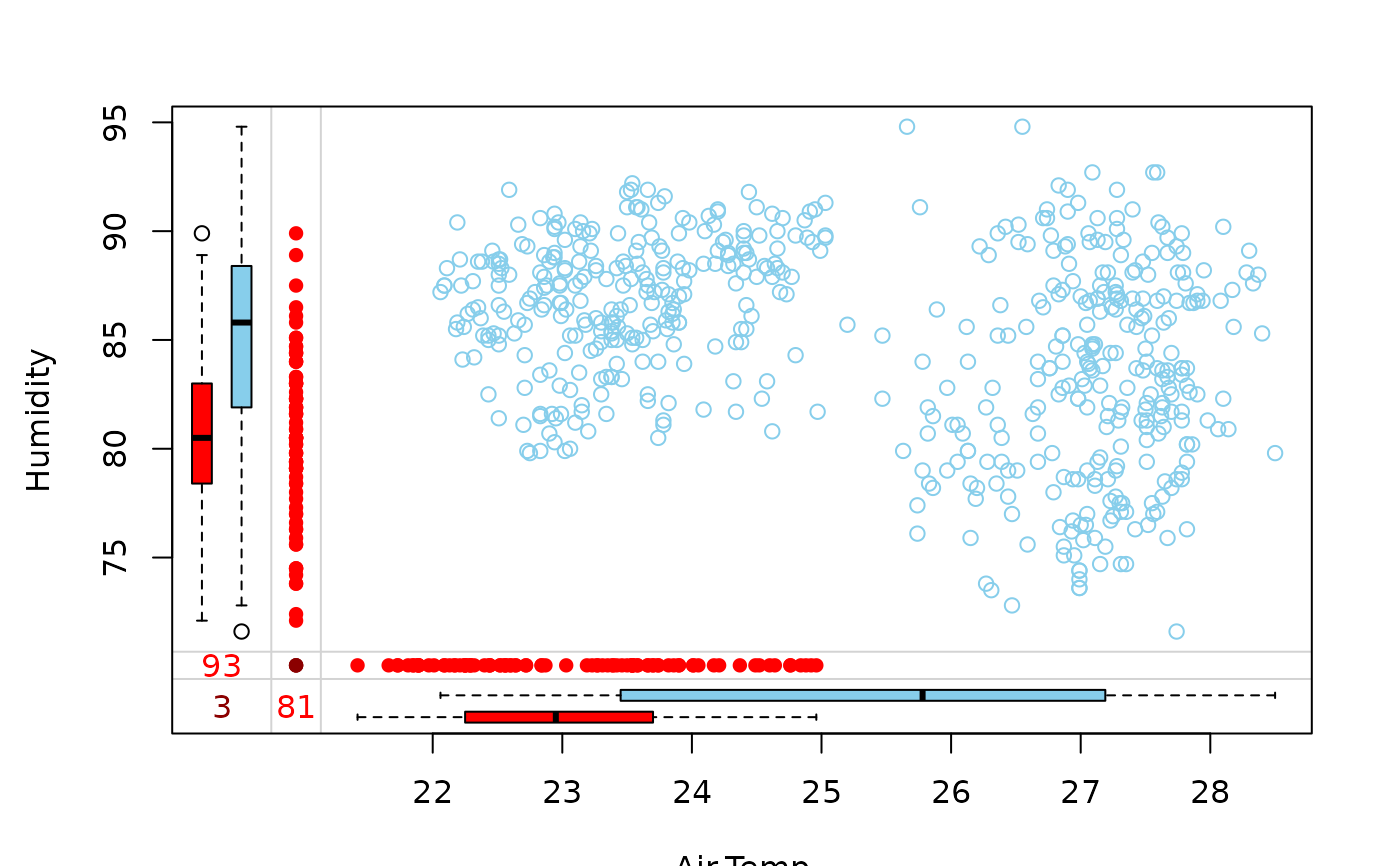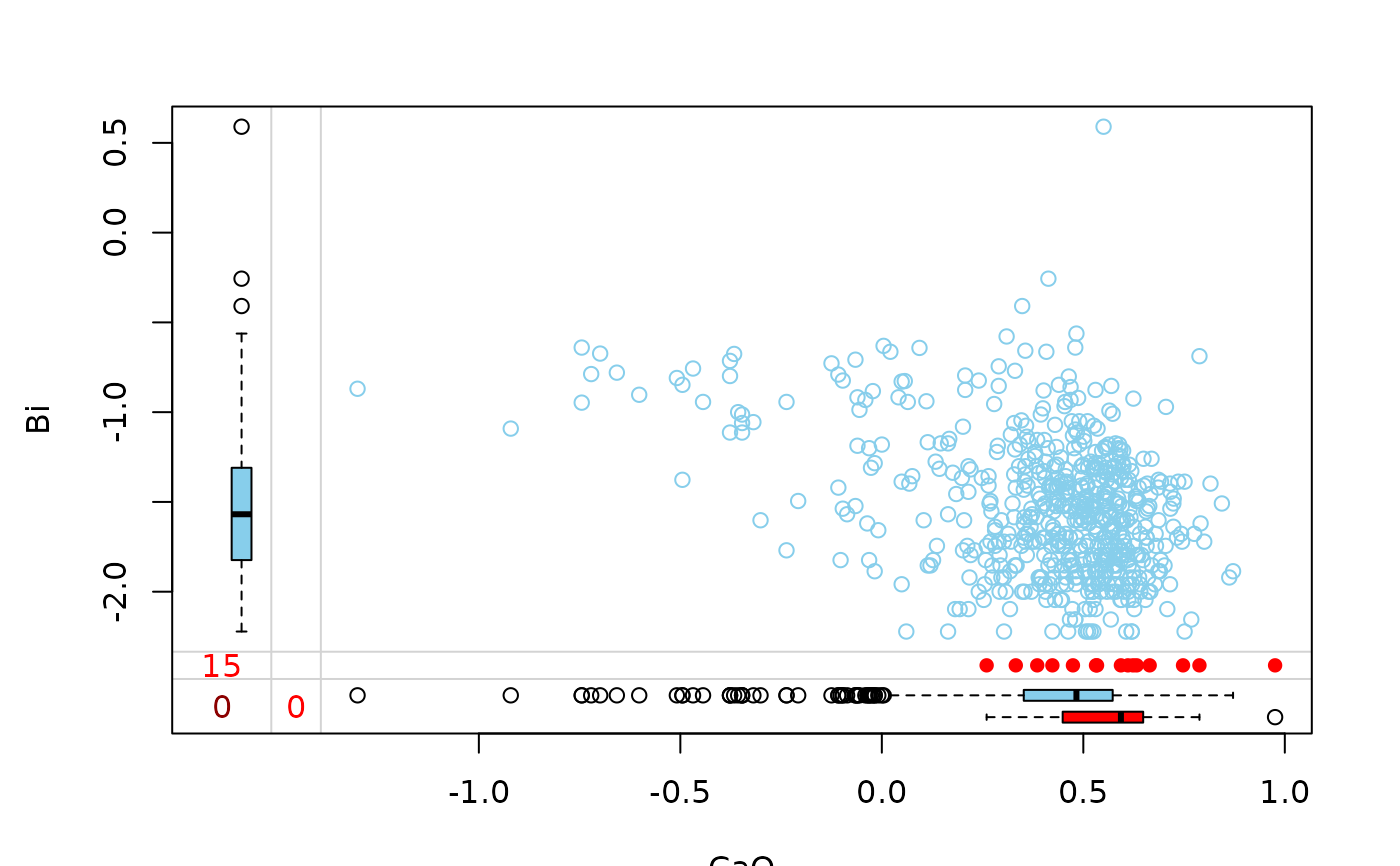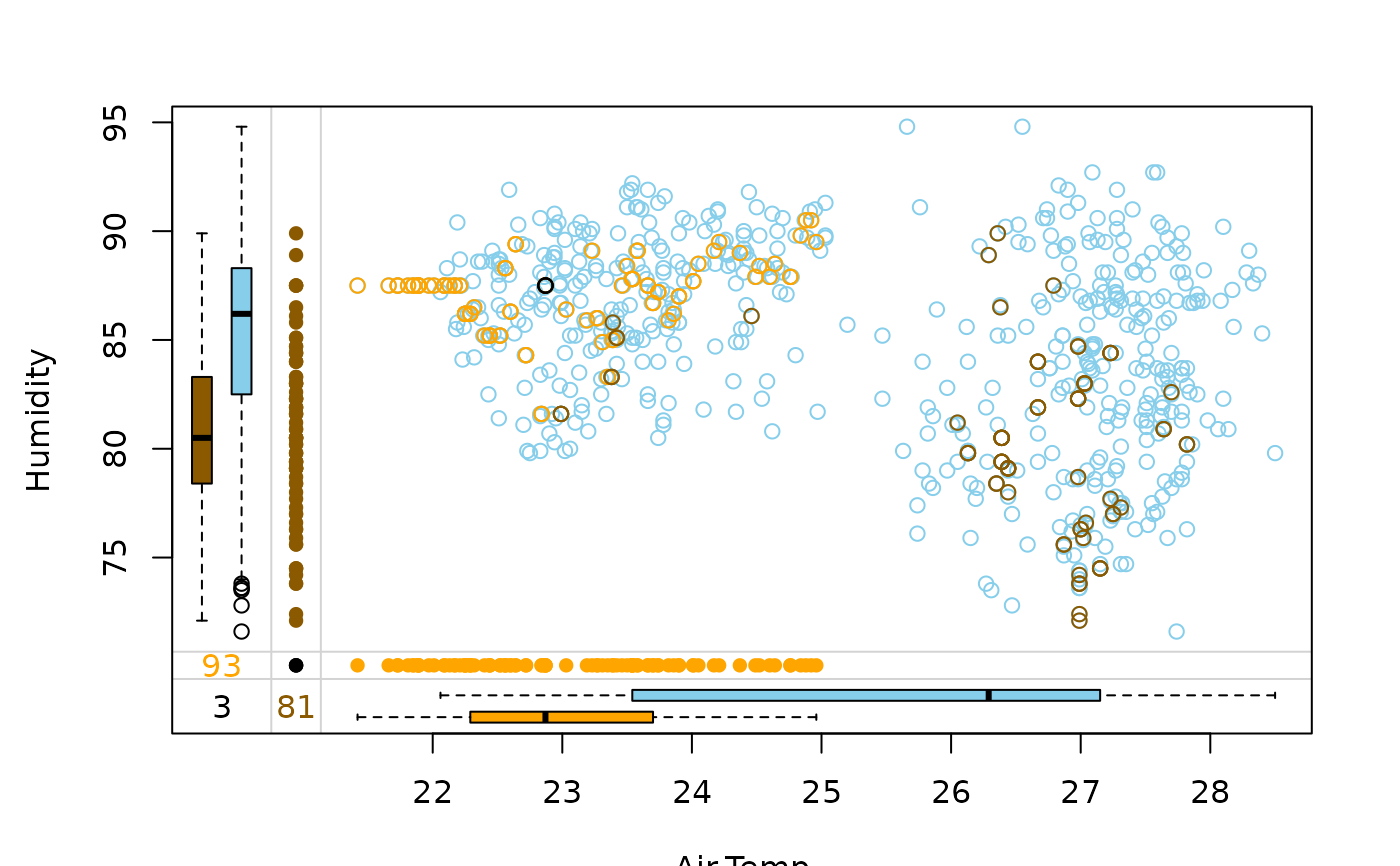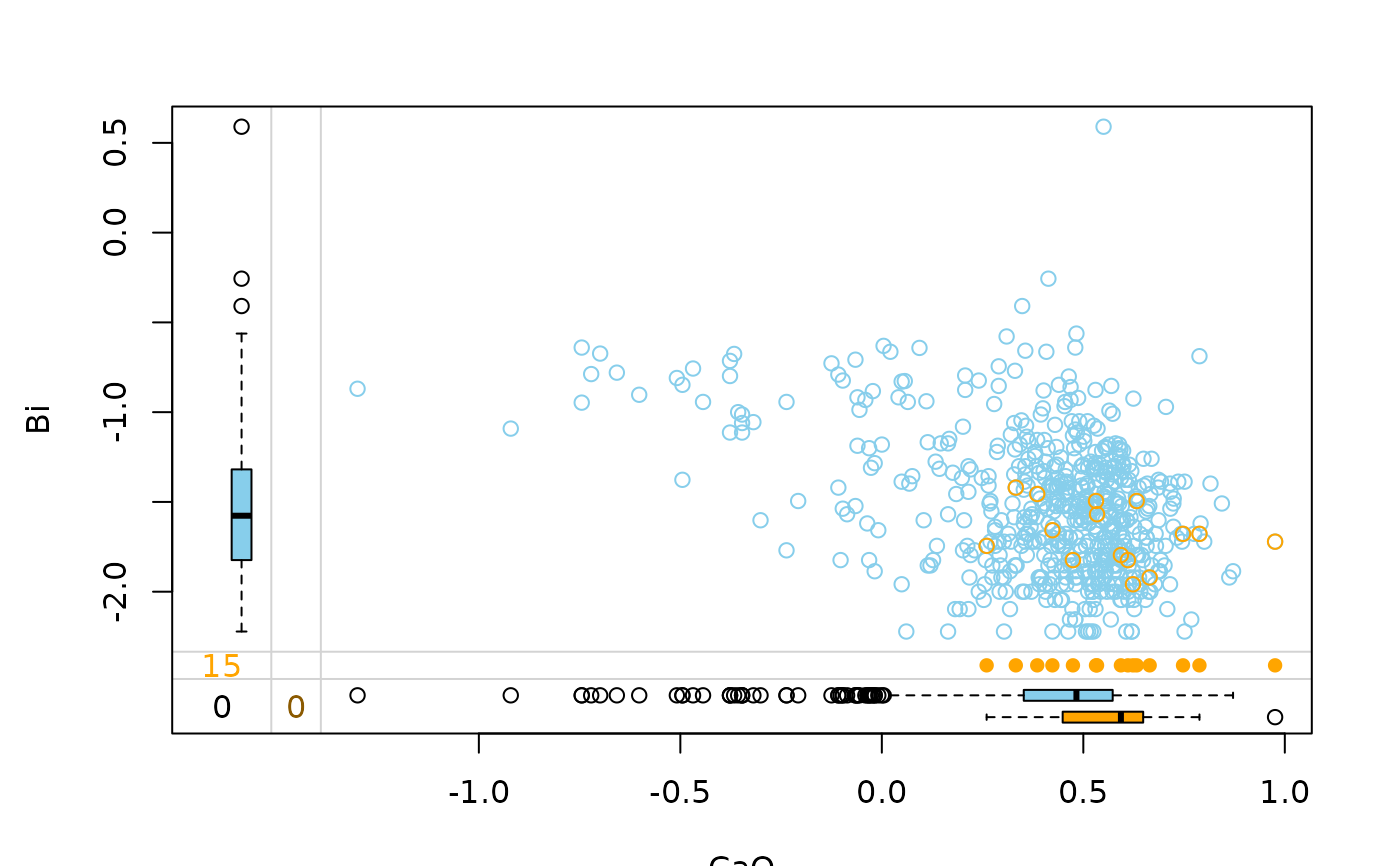In addition to a standard scatterplot, information about missing/imputed values is shown in the plot margins. Furthermore, imputed values are highlighted in the scatterplot.

marginplot(
x,
delimiter = NULL,
col = c("skyblue", "red", "red4", "orange", "orange4"),
alpha = NULL,
pch = c(1, 16),
cex = par("cex"),
numbers = TRUE,
cex.numbers = par("cex"),
zeros = FALSE,
xlim = NULL,
ylim = NULL,
main = NULL,
sub = NULL,
xlab = NULL,
ylab = NULL,
ann = par("ann"),
axes = TRUE,
frame.plot = axes,
...
)

## Arguments

x

a matrix or data.frame with two columns.

delimiter

a character-vector to distinguish between variables and imputation-indices for imputed variables (therefore, x needs to have colnames()). If given, it is used to determine the corresponding imputation-index for any imputed variable (a logical-vector indicating which values of the variable have been imputed). If such imputation-indices are found, they are used for highlighting and the colors are adjusted according to the given colors for imputed variables (see col).

col

a vector of length five giving the colors to be used in the plot. The first color is used for the scatterplot and the boxplots for the available data. In case of missing values, the second color is taken for the univariate scatterplots and boxplots for missing values in one variable and the third for the frequency of missing/imputed values in both variables (see ‘Details’). Otherwise, in case of imputed values, the fourth color is used for the highlighting, the frequency, the univariate scatterplot and the boxplots of mputed values in the first variable and the fifth color for the same applied to the second variable. A black color is used for the highlighting and the frequency of imputed values in both variables instead. If only one color is supplied, it is used for the bivariate and univariate scatterplots and the boxplots for missing/imputed values in one variable, whereas the boxplots for the available data are transparent. Else if two colors are supplied, the second one is recycled.

alpha

a numeric value between 0 and 1 giving the level of transparency of the colors, or NULL. This can be used to prevent overplotting.

pch

a vector of length two giving the plot symbols to be used for the scatterplot and the univariate scatterplots. If a single plot character is supplied, it is used for the scatterplot and the default value will be used for the univariate scatterplots (see ‘Details’).

cex

the character expansion factor to be used for the bivariate and univariate scatterplots.

numbers

a logical indicating whether the frequencies of missing/imputed values should be displayed in the lower left of the plot (see ‘Details’).

cex.numbers

the character expansion factor to be used for the frequencies of the missing/imputed values.

zeros

a logical vector of length two indicating whether the variables are semi-continuous, i.e., contain a considerable amount of zeros. If TRUE, only the non-zero observations are used for drawing the respective boxplot. If a single logical is supplied, it is recycled.

xlim, ylim

axis limits.

main, sub

main and sub title.

xlab, ylab

axis labels.

ann

a logical indicating whether plot annotation (main, sub, xlab, ylab) should be displayed.

axes

a logical indicating whether both axes should be drawn on the plot. Use graphical parameter "xaxt" or "yaxt" to suppress only one of the axes.

frame.plot

a logical indicating whether a box should be drawn around the plot.

...

further graphical parameters to be passed down (see graphics::par()).

## Details

Boxplots for available and missing/imputed data, as well as univariate scatterplots for missing/imputed values in one variable are shown in the plot margins.

Imputed values in either of the variables are highlighted in the scatterplot.

Furthermore, the frequencies of the missing/imputed values can be displayed by a number (lower left of the plot). The number in the lower left corner is the number of observations that are missing/imputed in both variables.

## Note

Some of the argument names and positions have changed with versions 1.3 and 1.4 due to extended functionality and for more consistency with other plot functions in VIM. For back compatibility, the argument cex.text can still be supplied to ...{} and is handled correctly. Nevertheless, it is deprecated and no longer documented. Use cex.numbers instead.

scattMiss()

Other plotting functions: aggr(), barMiss(), histMiss(), marginmatrix(), matrixplot(), mosaicMiss(), pairsVIM(), parcoordMiss(), pbox(), scattJitt(), scattMiss(), scattmatrixMiss(), spineMiss()

## Author

Andreas Alfons, Matthias Templ, modifications by Bernd Prantner

## Examples



data(tao, package = "VIM")
data(chorizonDL, package = "VIM")
## for missing values
marginplot(tao[,c("Air.Temp", "Humidity")])marginplot(log10(chorizonDL[,c("CaO", "Bi")]))## for imputed values
marginplot(kNN(tao[,c("Air.Temp", "Humidity")]), delimiter = "_imp")marginplot(kNN(log10(chorizonDL[,c("CaO", "Bi")])), delimiter = "_imp")